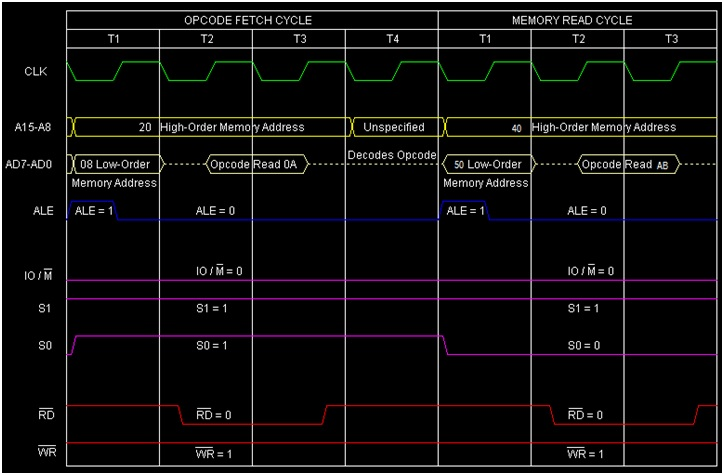# Instruction type LDAX rp in 8085 Microprocessor

In 8085 Instruction set, LDAX is a mnemonic that stands for LoaD Accumulator from memory pointed by eXtended register pair denoted as “rp” in the instruction. This instruction uses register indirect addressing for specifying the data. It occupies only 1-Byte in the memory.

This rp can be either BC register pair represented by B or DE register pair represented by D.Note that LDAX H is not provided in 8085 instruction set. This is because, LDAX H is the same as MOV A, M in its function.

Mnemonics, Operand Opcode(in HEX) Bytes
LDAX B 0A 1
LDAX D 1A 1

Let us consider, LDAX B as an example instruction of this type. As It is a 1-Byte instruction so it will occupy 1-Byte in the memory. Let us suppose that the initial content of BC register pair is 4050H and at memory location 4050H is having the 8-bit content of ABH. Let the Accumulator initial content is CDH. So after execution of the instruction the Accumulator will have the updated content of ABH. Let us consider the tracing table against execution of this instruction below −

Before After
(BC) 4050H 4050H
(4050H) ABH ABH
A CDH ABH